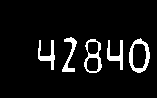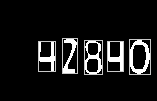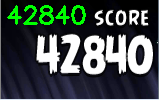#### OpenCV实现最简单的数字识别

Home / C++ 2016-10-19 5656

1. 学习分类器

```train_X = cvCreateMat(sample_num * class_num, size * size, CV_32FC1);
train_Y = cvCreateMat(sample_num * class_num, 1, CV_32FC1);
for(i = 0; i < class_num; i++){
for(j = 0; j < sample_num; j++){
pimage = preprocessing(src_image, size, size);
...
cvGetRow(train_X, &row, i * sample_num + j);
row_vec = cvReshape(&data, &mathdr, 0, 1);
cvCopy(row_vec, &row, NULL);
...
cvGetRow(train_Y, &row, i * sample_num + j);
cvSet(&row, cvRealScalar(i));
}
}```

```IplImage preprocessing(IplImage* img, int w, int h){
...
bb = findBoundingBox(img);
cvGetSubRect(img, &data, cvRect(bb.x, bb.y, bb.width, bb.height));
size = (bb.width > bb.height) ? bb.width : bb.height;

res = cvCreateImage(cvSize(size, size), 8, 1);
x = floor((float)(size - bb.width) / 2.0f);
y = floor((float)(size - bb.height) / 2.0f);
cvGetSubRect(res, &subdata, cvRect((int)x, (int)y, bb.width, bb.height));
cvCopy(&data, &subdata, NULL);
ret = cvCreateImage(cvSize(w, h), 8, 1);
cvResize(res, ret, CV_INTER_NN);
return *ret;
}```

`knn = new CvKNearest(train_X, train_Y, 0, false, K); `

2. 图像预处理

```cvtColor(input, out_img, CV_BGR2GRAY);
threshold(out_img, out_img, 0, 255, CV_THRESH_OTSU + CV_THRESH_BINARY);
...
erode(out_img, out_img, elem); ```3. 图像分割

```vector< vector< Point> > contours;
findContours(contour_img, contours, CV_RETR_EXTERNAL, CV_CHAIN_APPROX_NONE);

vector<vector<Point> >::iterator it = contours.begin();
while (it!=contours.end()) {
RotatedRect rect = minAreaRect(Mat(*it));
if(verifyRect(rect)){
++it; // A valid rectangle found
} else {
it= contours.erase(it);
}
}

...
vector<Rect> boundRect(contours.size());
for (int i = 0; i < contours.size(); ++i) {
Scalar color = Scalar(200, 200, 200);
boundRect[i] = boundingRect(Mat(contours[i]));
rectangle(out_img, boundRect[i].tl(), boundRect[i].br(), color, 0.2, 8, 0);

CvRect roi = CvRect(boundRect[i]);
IplImage orig = out_img;
IplImage *res = cvCreateImage(cvSize(roi.width, roi.height), orig.depth, orig.nChannels);
cvSetImageROI(&orig, roi);
cvCopy(&orig, res);
cvResetImageROI(&orig);

IplImage *bininv_img;
bininv_img = cvCreateImage(cvSize(128, 128), IPL_DEPTH_8U, 1);
cvResize(res, bininv_img);
cvThreshold(bininv_img, bininv_img, 100, 255, CV_THRESH_BINARY_INV);

int ret = do_ocr(bininv_img);
res_elem elem;
elem.num = ret;
elem.xpos = boundRect[i].tl().x;
res_vec.push_back(elem);
...
}  ```4. 应用分类器

```int do_ocr(IplImage *img)
{
...
pimage = preprocessing(img, size, size);

...
cvGetSubRect(pimage, &data, cvRect(0, 0, size, size));
CvMat mathdr, *vec;
vec = cvReshape(&data, &mathdr, 0, 1);
ret = knn->find_nearest(vec, K, 0, 0, nearest, 0);
return (int)ret;
}```

5. 后期处理

```sort(res_vec.begin(), res_vec.end(), sort_func);
int j, num = 0;
for (j = 0; j < res_vec.size(); ++j) {
num = num * 10 + res_vec[j].num;
}
char resbuf;
sprintf(resbuf, "%d", num);
putText(show_img, resbuf, Point(OUTPUT_X, OUTPUT_Y), FONT_HERSHEY_SIMPLEX, 0.8, Scalar(0, 255, 0), 2);
imshow("show", show_img);```